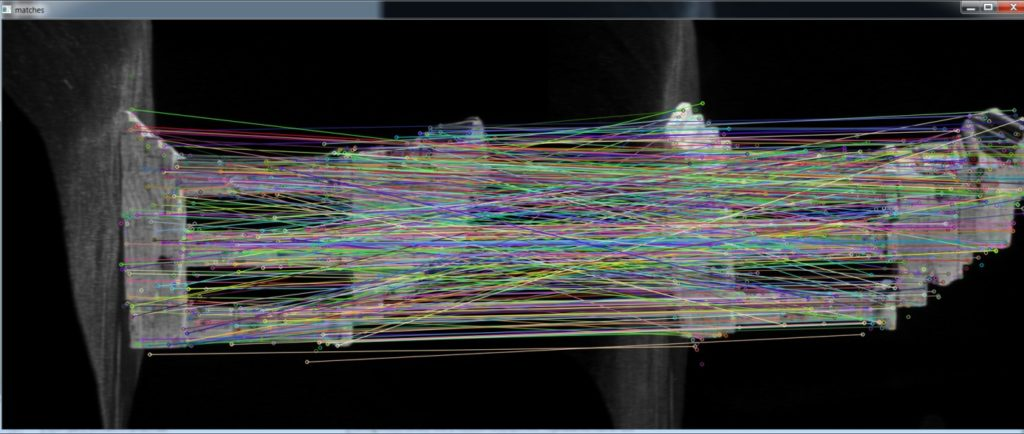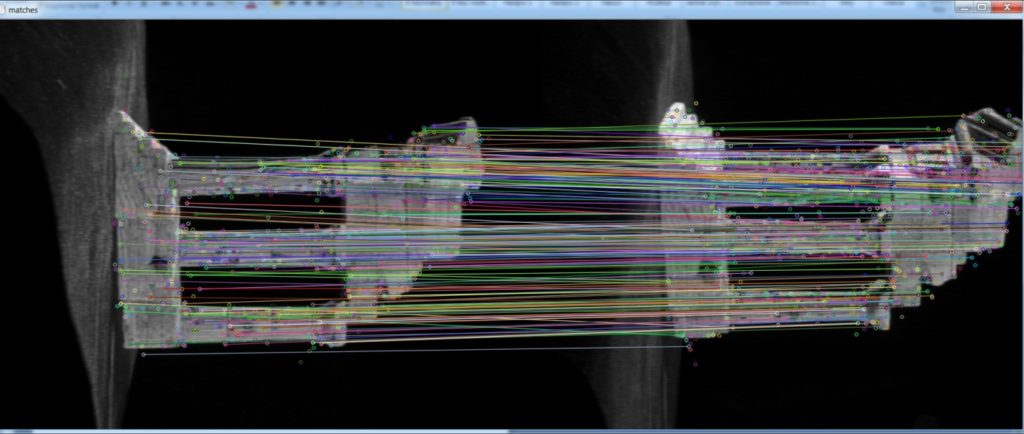Posted on

# Camera trackingMartin Volovar

Camera tracking is used in visual effects to synchronize movement and rotation between real and virtual camera .This article deals with obtaining rotation and translation from two images and trying to reconstruct scene.

1. First we need find keypoints on both images:
```SurfFeatureDetector detector(400);
vector<KeyPoint> keypoints1, keypoints2, findKeypoints;
detector.detect(img1, keypoints1);
detector.detect(img2, keypoints2);

SurfDescriptorExtractor extractor;
extractor.compute(img1, keypoints1, descriptors1);
extractor.compute(img2, keypoints2, descriptors2);
```
2. Then we need find matches between keypoints from first and second image:
```cv::BFMatcher matcher(cv::NORM_L2, true);
vector<DMatch> matches;
matcher.match(descriptors1, descriptors2, matches);
```3. Some keypoints are wrong so we use filtration:
```x = ABS(x);
y = ABS(y);

if (x < x_threshold && y < y_threshold)
status[i] = 1;
else
status[i] = 0;
```4. After that we can find dependency using FM:
```Mat FM = findFundamentalMat(keypointsPosition1, keypointsPosition2, FM_RANSAC, 1., 0.99, status);
```

we can obtain essential matrix using camera internal parameters (K matrix):

```Mat E = K. t() * FM * K;
```
5. Using singular value decomposition we can extract camera rotation and translation:
```SVD svd(E, SVD::MODIFY_A) ;
Mat svd_u = svd. u;
Mat svd_vt = svd. vt;
Mat svd_w = svd. w;
Matx33d W(0, -1, 0,
1, 0, 0,
0, 0, 1) ;

Mat R = svd_u * Mat(W) * svd_vt;
Mat_<double> t = svd_u. col(2) ;
```

Rotation have two solutions (R = U*W*VT or R = U*WT*VT), so we check if camera has right direction:

```double *R_D = (double*) R.data;
if (R_D < 0.0)
R = svd_u * Mat(W.t()) * svd_vt;
```

To construct rays we need inverse camera matrix (R|t):

```Mat Cam(4, 4, CV_64F, Cam_D);
Mat Cam_i = Cam.inv();
```

Both lines have one point in camera center:

```Line l0, l1;
l0.pos.x = 0.0;
l0.pos.y = 0.0;
l0.pos.z = 0.0;

l1.pos.x = Cam_iD;
l1.pos.y = Cam_iD;
l1.pos.z = Cam_iD;
```

Other point is calculated via projection plane.
Then we can construct rays and find intersection from each keypoint:

```getNearestPointBetweenTwoLines(pointCloud[j], l0, l1, k);
```